Latest Banking jobs   »

# Quantitative Aptitude Quiz For NABARD Grade A 2022- 13th August

Directions (1-5): In the given questions, two quantities are given, one as ‘Quantity I’ and another as ‘Quantity II’. You have to determine relationship between two quantities and choose the appropriate option:

Q1. Quantity I: x²+16x+63=0
Quantity II: x²+25x+144=0
(a) Quantity I > Quantity II
(b) Quantity I < Quantity II
(c) Quantity I ≥ Quantity II
(d) Quantity I ≤ Quantity II
(e) Quantity I = Quantity II or No relation

Q2. Quantity I: 3x²+22x+40=0
Quantity II: x²-16x-36=0
(a) Quantity I > Quantity II
(b) Quantity I < Quantity II
(c) Quantity I ≥ Quantity II
(d) Quantity I ≤ Quantity II
(e) Quantity I = Quantity II or No relation

Q3. Quantity I: x²=961
Quantity II: x²-36x+155=0
(a) Quantity I > Quantity II
(b) Quantity I < Quantity II
(c) Quantity I ≥ Quantity II
(d) Quantity I ≤ Quantity II
(e) Quantity I = Quantity II or No relation

Q4. Quantity I: x²+16x+6=72-3x
Quantity II: x²+13x-96=6+2x
(a) Quantity I > Quantity II
(b) Quantity I < Quantity II
(c) Quantity I ≥ Quantity II
(d) Quantity I ≤ Quantity II
(e) Quantity I = Quantity II or No relation

Q5. Quantity I: x²+29x-210=0
Quantity II: x²-21x+104=0
(a) Quantity I > Quantity II
(b) Quantity I < Quantity II
(c) Quantity I ≥ Quantity II
(d) Quantity I ≤ Quantity II
(e) Quantity I = Quantity II or No relation

Directions (6-10): Study the given passage carefully and answer the questions.
The total population of city X is 7100 out of which 24% population are below poverty line. And total population of city X is 11¼ % less than total population of city Z and 25% of population in city Z is below poverty line. Population other than below poverty line in city L is 160 more than population of city X which are not below poverty line.
Average population of Y and K is equal to average population of city X and Z & population of Y is 150% of population of K. Ratio of BPL in city Z to city K is 2 : 3. 20% of total population are BPL in city Y and Z. BPL in city L is equal to BPL in city X.

Q6. Average BPL population in city X and Y is how much more/less than average BPL population in city K and L ?
(a) 794
(b) 824
(c) 848
(d) 764
(e) None of these

Q7. Average population in city K and L is what percent of total population in city Z ?
(a) 93%
(b) 73%
(c) 83.125%
(d) 87.50%
(e) 78.625%

Q8. In another city ‘A’, BPL population is half of the population other than BPL in city K which is 25% of total population. Then find total population in city A ?
(a) 6820
(b) 6080
(c) 6240
(d) None of these
(e) 6040

Q9. What is the average BPL population in all the cities ?
(a) 1924
(b) None of these
(c) 1884
(d) 1724
(e) 1964

Q10. What is the ratio of BPL population in city Y to city L ?
(a) 426 : 353
(b) 353 : 426
(c) 351 : 425
(d) None of these
(e) 353 : 428

Directions (11-15): Study the table carefully and answer the questions.
Table given below shows percentage of books sold of 3 different publications by five different seller in a month.
Note: Books are sold by three publication only.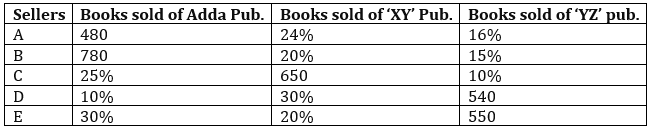Q11. Books sold by seller B of XY and YZ pub. Together is how much more/less than books sold by E of Adda & YZ publications together ?
(a) 360
(b) None of these
(c) 380
(d) 420
(e) 460

Q12. Books sold by seller C of Adda & XY together is what percent of total books sold by seller D ?
(a) 100%
(b) 80%
(c) None of these
(d) 150%
(e) 120%

Q13. What is average number of books sold by all sellers of Adda publication ?
(a) 392
(b) 386
(c) 406
(d) None of these
(e) 414

Q14. If selling price of each book of Adda publication sold by seller C is Rs. 250 and selling price of each book of XY publication sold by seller D is Rs. 220. Then find the difference in selling price of books of Adda publication sold by C and XY publication sold by D ?
(a) Rs. 4500
(b) Rs. 2900
(c) Rs. 3600
(d) Rs. 3100
(e) Rs. 4200

Q15. If profit made on each book sold by seller E is Rs. 44. Then find profit percent of each book sold by seller E ? (given that selling price of each book is Rs. 264)
(a) 22%
(b) 25%
(c) 20%
(d) 15%
(e) 30%

Solutions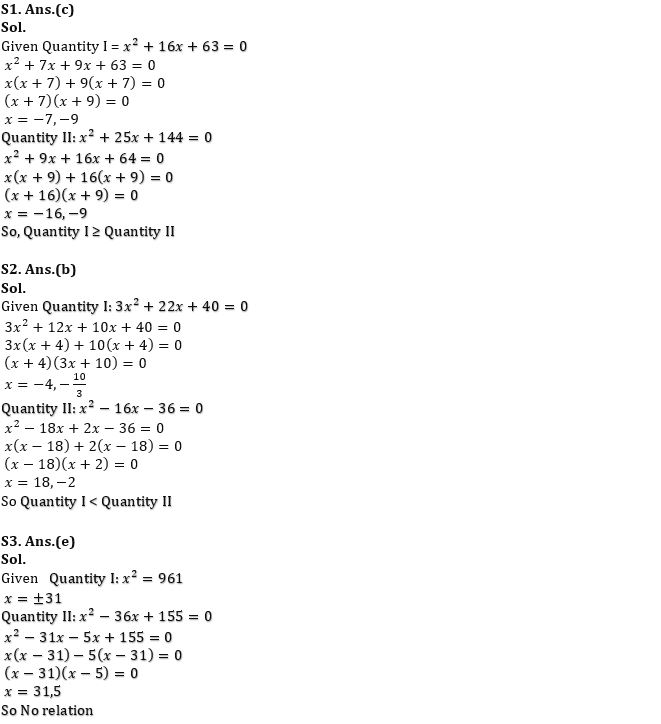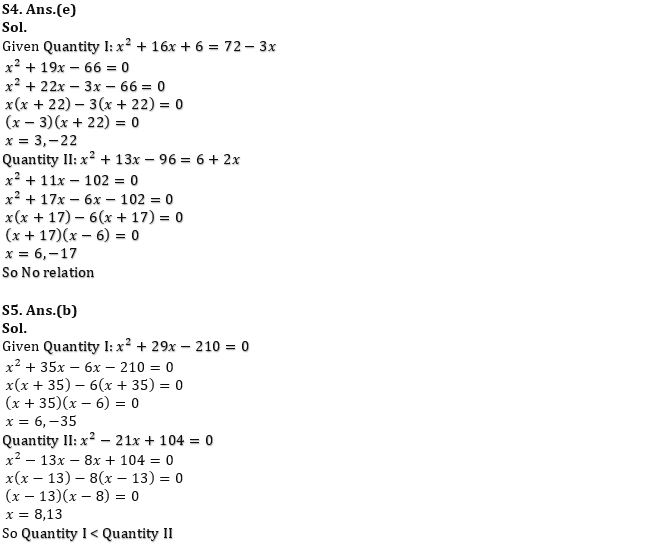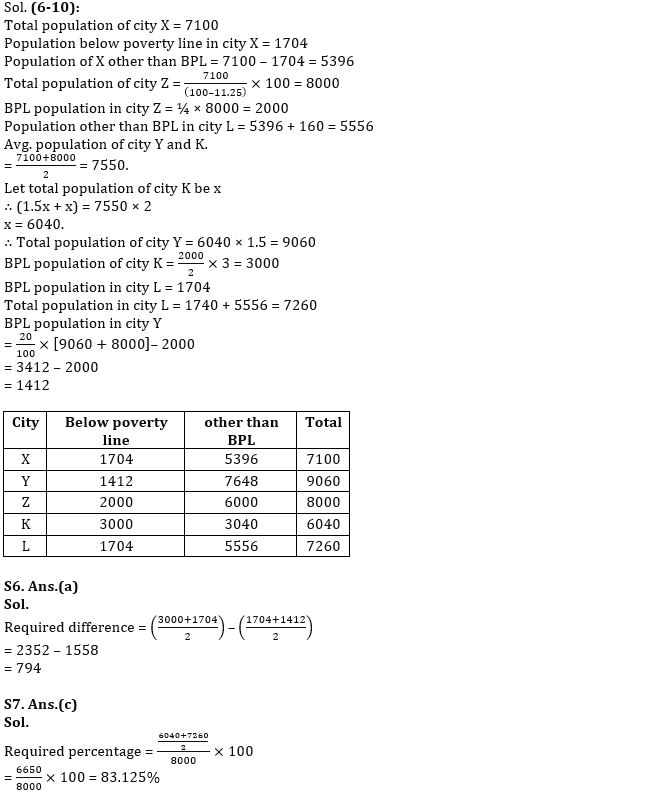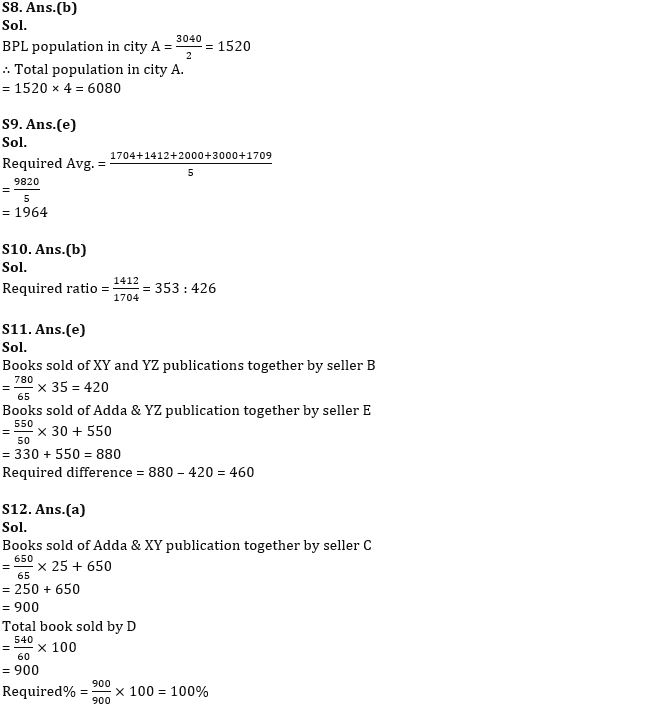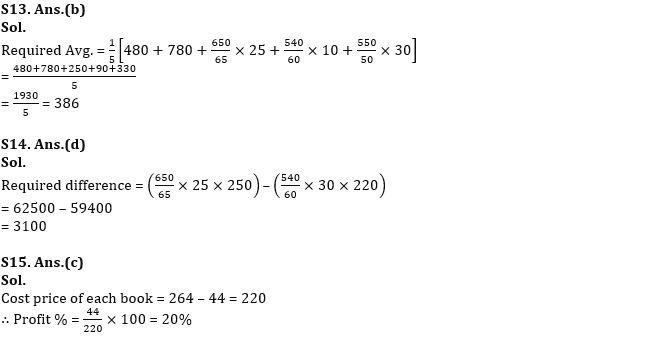#### Congratulations!Download Hindu Review of October 2021: Free PDFIncorrect details? Fill the form again here

Download Hindu Review of October 2021: Free PDF

Thank You, Your details have been submitted we will get back to you.
•General Awareness Quiz Series 2022: 4th ...
•World Animal Welfare Day 2022, Theme, Hi...
•GST revenue collected in the month of Se...
•American Express ties up with Nasscom to...
•Active credit cards drop 2.3 million in ...
•Quantitative Aptitude Quiz For SBI PO Pr...